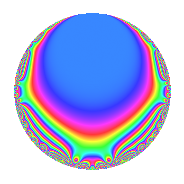# Properties

 Label 4014.2.a.mLevel 4014 Weight 2 Character orbit 4014.a Self dual Yes Analytic conductor 32.052 Analytic rank 1 Dimension 3 CM No Inner twists 1

# Related objects

## Newspace parameters

 Level: $$N$$ = $$4014 = 2 \cdot 3^{2} \cdot 223$$ Weight: $$k$$ = $$2$$ Character orbit: $$[\chi]$$ = 4014.a (trivial)

## Newform invariants

 Self dual: Yes Analytic conductor: $$32.0519513713$$ Analytic rank: $$1$$ Dimension: $$3$$ Coefficient field: $$\Q(\zeta_{18})^+$$ Coefficient ring: $$\Z[a_1, \ldots, a_{5}]$$ Coefficient ring index: $$1$$ Fricke sign: $$1$$ Sato-Tate group: $\mathrm{SU}(2)$

## $q$-expansion

Coefficients of the $$q$$-expansion are expressed in terms of a root $$\beta$$ of the polynomial $$x^{3} - 3 x - 1$$. We also show the integral $$q$$-expansion of the trace form.

 $$f(q)$$ $$=$$ $$q - q^{2} + q^{4} + ( 1 + \beta ) q^{5} + ( 1 + \beta - \beta^{2} ) q^{7} - q^{8} +O(q^{10})$$ $$q - q^{2} + q^{4} + ( 1 + \beta ) q^{5} + ( 1 + \beta - \beta^{2} ) q^{7} - q^{8} + ( -1 - \beta ) q^{10} + ( 2 - \beta - \beta^{2} ) q^{11} + ( -7 - \beta + 2 \beta^{2} ) q^{13} + ( -1 - \beta + \beta^{2} ) q^{14} + q^{16} + ( 2 - 3 \beta ) q^{17} + ( 1 - \beta^{2} ) q^{19} + ( 1 + \beta ) q^{20} + ( -2 + \beta + \beta^{2} ) q^{22} + ( 4 + 3 \beta - \beta^{2} ) q^{23} + ( -4 + 2 \beta + \beta^{2} ) q^{25} + ( 7 + \beta - 2 \beta^{2} ) q^{26} + ( 1 + \beta - \beta^{2} ) q^{28} + ( -9 - \beta + 5 \beta^{2} ) q^{29} + ( -9 - 4 \beta + 3 \beta^{2} ) q^{31} - q^{32} + ( -2 + 3 \beta ) q^{34} -\beta q^{35} + ( 12 + 3 \beta - 7 \beta^{2} ) q^{37} + ( -1 + \beta^{2} ) q^{38} + ( -1 - \beta ) q^{40} + ( 8 + 4 \beta - 3 \beta^{2} ) q^{41} + ( -6 + 3 \beta^{2} ) q^{43} + ( 2 - \beta - \beta^{2} ) q^{44} + ( -4 - 3 \beta + \beta^{2} ) q^{46} + ( -7 - \beta + 6 \beta^{2} ) q^{47} + ( -8 - 3 \beta + 2 \beta^{2} ) q^{49} + ( 4 - 2 \beta - \beta^{2} ) q^{50} + ( -7 - \beta + 2 \beta^{2} ) q^{52} + ( 5 - 6 \beta - \beta^{2} ) q^{53} + ( 1 - 2 \beta - 2 \beta^{2} ) q^{55} + ( -1 - \beta + \beta^{2} ) q^{56} + ( 9 + \beta - 5 \beta^{2} ) q^{58} + ( 2 + 3 \beta - 2 \beta^{2} ) q^{59} + ( -7 - 3 \beta + \beta^{2} ) q^{61} + ( 9 + 4 \beta - 3 \beta^{2} ) q^{62} + q^{64} + ( -5 - 2 \beta + \beta^{2} ) q^{65} + ( -17 - 5 \beta + 8 \beta^{2} ) q^{67} + ( 2 - 3 \beta ) q^{68} + \beta q^{70} -3 q^{71} + ( 3 \beta - 2 \beta^{2} ) q^{73} + ( -12 - 3 \beta + 7 \beta^{2} ) q^{74} + ( 1 - \beta^{2} ) q^{76} + ( 2 + 2 \beta - \beta^{2} ) q^{77} + ( 6 + \beta - 3 \beta^{2} ) q^{79} + ( 1 + \beta ) q^{80} + ( -8 - 4 \beta + 3 \beta^{2} ) q^{82} + ( 1 - 6 \beta - \beta^{2} ) q^{83} + ( 2 - \beta - 3 \beta^{2} ) q^{85} + ( 6 - 3 \beta^{2} ) q^{86} + ( -2 + \beta + \beta^{2} ) q^{88} + ( -3 - 7 \beta + \beta^{2} ) q^{89} + ( -4 - \beta + 2 \beta^{2} ) q^{91} + ( 4 + 3 \beta - \beta^{2} ) q^{92} + ( 7 + \beta - 6 \beta^{2} ) q^{94} + ( -2 \beta - \beta^{2} ) q^{95} + ( -3 - 3 \beta^{2} ) q^{97} + ( 8 + 3 \beta - 2 \beta^{2} ) q^{98} +O(q^{100})$$ $$\operatorname{Tr}(f)(q)$$ $$=$$ $$3q - 3q^{2} + 3q^{4} + 3q^{5} - 3q^{7} - 3q^{8} + O(q^{10})$$ $$3q - 3q^{2} + 3q^{4} + 3q^{5} - 3q^{7} - 3q^{8} - 3q^{10} - 9q^{13} + 3q^{14} + 3q^{16} + 6q^{17} - 3q^{19} + 3q^{20} + 6q^{23} - 6q^{25} + 9q^{26} - 3q^{28} + 3q^{29} - 9q^{31} - 3q^{32} - 6q^{34} - 6q^{37} + 3q^{38} - 3q^{40} + 6q^{41} - 6q^{46} + 15q^{47} - 12q^{49} + 6q^{50} - 9q^{52} + 9q^{53} - 9q^{55} + 3q^{56} - 3q^{58} - 6q^{59} - 15q^{61} + 9q^{62} + 3q^{64} - 9q^{65} - 3q^{67} + 6q^{68} - 9q^{71} - 12q^{73} + 6q^{74} - 3q^{76} + 3q^{80} - 6q^{82} - 3q^{83} - 12q^{85} - 3q^{89} + 6q^{92} - 15q^{94} - 6q^{95} - 27q^{97} + 12q^{98} + O(q^{100})$$

## Embeddings

For each embedding $$\iota_m$$ of the coefficient field, the values $$\iota_m(a_n)$$ are shown below.

For more information on an embedded modular form you can click on its label.

Label $$\iota_m(\nu)$$ $$a_{2}$$ $$a_{3}$$ $$a_{4}$$ $$a_{5}$$ $$a_{6}$$ $$a_{7}$$ $$a_{8}$$ $$a_{9}$$ $$a_{10}$$
1.1
 −1.53209 −0.347296 1.87939
−1.00000 0 1.00000 −0.532089 0 −2.87939 −1.00000 0 0.532089
1.2 −1.00000 0 1.00000 0.652704 0 0.532089 −1.00000 0 −0.652704
1.3 −1.00000 0 1.00000 2.87939 0 −0.652704 −1.00000 0 −2.87939
 $$n$$: e.g. 2-40 or 990-1000 Significant digits: Format: Complex embeddings Normalized embeddings Satake parameters Satake angles

## Inner twists

This newform does not admit any (nontrivial) inner twists.

## Atkin-Lehner signs

$$p$$ Sign
$$2$$ $$1$$
$$3$$ $$-1$$
$$223$$ $$-1$$

## Hecke kernels

This newform can be constructed as the intersection of the kernels of the following linear operators acting on $$S_{2}^{\mathrm{new}}(\Gamma_0(4014))$$:

 $$T_{5}^{3} - 3 T_{5}^{2} + 1$$ $$T_{7}^{3} + 3 T_{7}^{2} - 1$$ $$T_{11}^{3} - 9 T_{11} + 9$$